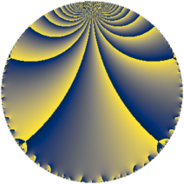# Properties

 Label 252.4.eLevel $252$ Weight $4$ Character orbit 252.e Rep. character $\chi_{252}(71,\cdot)$ Character field $\Q$ Dimension $36$ Newform subspaces $1$ Sturm bound $192$ Trace bound $0$

# Learn more

## Defining parameters

 Level: $$N$$ $$=$$ $$252 = 2^{2} \cdot 3^{2} \cdot 7$$ Weight: $$k$$ $$=$$ $$4$$ Character orbit: $$[\chi]$$ $$=$$ 252.e (of order $$2$$ and degree $$1$$) Character conductor: $$\operatorname{cond}(\chi)$$ $$=$$ $$12$$ Character field: $$\Q$$ Newform subspaces: $$1$$ Sturm bound: $$192$$ Trace bound: $$0$$

## Dimensions

The following table gives the dimensions of various subspaces of $$M_{4}(252, [\chi])$$.

Total New Old
Modular forms 152 36 116
Cusp forms 136 36 100
Eisenstein series 16 0 16

## Trace form

 $$36q - 24q^{4} + O(q^{10})$$ $$36q - 24q^{4} + 264q^{10} - 468q^{16} + 444q^{22} - 900q^{25} - 84q^{28} - 432q^{34} - 264q^{37} + 1416q^{40} + 180q^{46} - 1764q^{49} + 2736q^{52} + 636q^{58} - 3960q^{61} + 1392q^{64} - 504q^{70} - 2520q^{76} + 1032q^{82} - 3144q^{85} + 2748q^{88} + 5496q^{94} + O(q^{100})$$

## Decomposition of $$S_{4}^{\mathrm{new}}(252, [\chi])$$ into newform subspaces

Label Dim. $$A$$ Field CM Traces $q$-expansion
$$a_2$$ $$a_3$$ $$a_5$$ $$a_7$$
252.4.e.a $$36$$ $$14.868$$ None $$0$$ $$0$$ $$0$$ $$0$$

## Decomposition of $$S_{4}^{\mathrm{old}}(252, [\chi])$$ into lower level spaces

$$S_{4}^{\mathrm{old}}(252, [\chi]) \cong$$ $$S_{4}^{\mathrm{new}}(12, [\chi])$$$$^{\oplus 4}$$$$\oplus$$$$S_{4}^{\mathrm{new}}(36, [\chi])$$$$^{\oplus 2}$$$$\oplus$$$$S_{4}^{\mathrm{new}}(84, [\chi])$$$$^{\oplus 2}$$